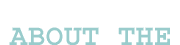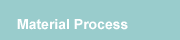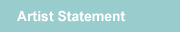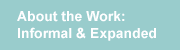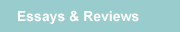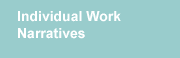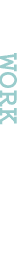Identity Sequence e Black I created a system. I defined a specific method for directly articulating the digits; I defined colors and a sequence for the colors; chose a number sequence; and followed the plan to create the work. I was thinking about: identity codes, internal patterns that code the individual, DNA, external codes, population, individuals and masses. I wanted to use a sequence large enough that a pattern would be revealed, a pattern that read as some sort of code, a blueprint that described something specific. Even if it was not clear what that thing was, I wanted the pattern to resonate a purpose. “Identity Sequence e Black” is based on the first 49 digits of the number e. I wanted a small section that seems to be cut away from a much larger one. Grid is constructed from 49 units in 7 rows by 7 columns. 7 is a prime number. Here the digits are articulated by two systems. Tonal gradation of the black where the darkest color value represents the 9 digit and no color represents 0. And the distance each unit pushes away from the wall, where units representing the 9 digit push 32” away from the wall and the 0s barely pooch up. The units are joined to form 3 vertical sections (2 columns, 3 columns, 2 columns). Can be shown as one solid grid or sections can be separated to accommodate limited linear footage or entry ways. A prime number is a positive integer that can only be divided by 1 and itself. Prime numbers stand out from a sea of other numbers as they are the multiplicative root of all other numbers. They are the beginning, yet there are an infinite number of them, they cannot be contained. Often, nature grows in primes. Brief definition of the number e e is a real number constant that appears in certain kinds of mathematics problems. Examples of such problems are those involving growth or decay, the statistical “bell curve”, the shape of a hanging cable, some problems of probability, some counting problems, the study of the distribution of prime numbers, and often shows up in calculus. Its value is approximately 2.718281828459045… and has been calculated to 869,894,101 decimal places. e is also the base of natural logarithms. e is a transcendental number. Transcendental number is not expressible as an integer or as the root or quotient of integers.